# Structured References

When working with tables in Excel, you can use structured references to make your formulas easier to understand.

For example, we have the following table.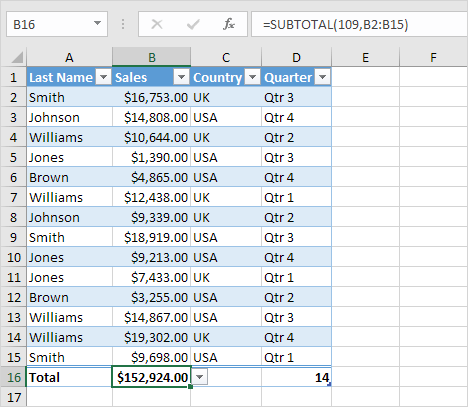1. Select cell E1, type Bonus, and press Enter. Excel automatically formats the column for you.2. Select cell E2 and type =0.02*[

3. A list of structured references (the columns) appears. Select Sales from the list.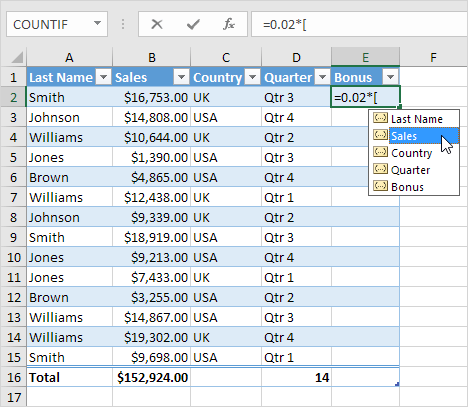4. Close with a square bracket and press Enter.

Result. Excel automatically copies the formula down the column for you.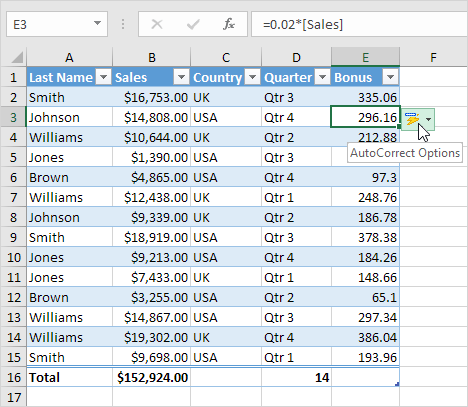Note: click AutoCorrect Options and click Undo Calculated Column to only insert the formula into cell E2.

To refer to this table in a formula, execute the following steps.

5. First, select a cell inside the table. Next, on the Design tab, in the Properties group, we can see that Table1 is the name of this table.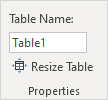6. Select cell E18 and enter the formula shown below.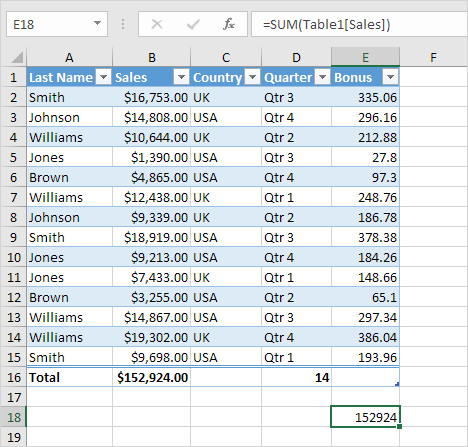Explanation: this formula calculates the sum of the Sales column. Enter =SUM(Table1[ and Excel will give you a list of structured references you can use. This formula updates automatically when you add or delete records.